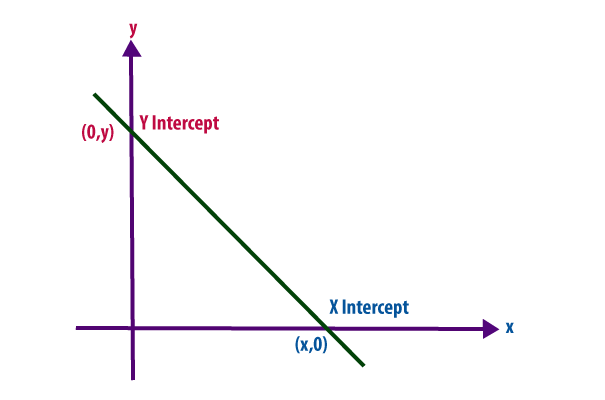# X and Y Intercept Formula

The graphical concept of x- and y-intercepts is pretty simple. The x-intercepts are where the graph crosses the x-axis, and the y-intercepts are where the graph crosses the y-axis. The X-intercept of a line gives the idea about the point which crosses the x-axis.

Same way, the y-intercept is a point at which the line crosses the y-axis. One can find out only one intercept at a time in a given equation.

#The x-intercept of a line is the point at which the line crosses the x axis. ( i.e. where the y value equals 0 )

$\large x-intercept = (x, 0)$

The y-intercept of a line is the point at which the line crosses the y axis. ( i.e. where the x value equals 0 )

$\large y-intercept = (0, y)$

### Solved Example of X and Y Intercept

Example: Find the x- and y-intercepts of

$$\begin{array}{l}25x^{^{2}}+4y^{^{2}}=9\end{array}$$

Solution
Use the formula of the intercepts to find X and Y Intercept separately.

x-intercept:
Substituting y = 0 for the x-intercept, so:

$$\begin{array}{l}25x^{^{2}}+4y^{^{2}}=9\\25x^{^{2}}+4\left (0\right)^{2}=9\\25x^{^{2}}+0=9\\x^{2}=\frac{9}{25}\\x^{2}=\pm \frac{3}{5}\end{array}$$

Then the x-intercepts are the points (

$$\begin{array}{l}\frac{3}{5}, 0\end{array}$$
) and (
$$\begin{array}{l}\frac{-3}{5}, 0\end{array}$$
).

y-intercept:
Substituting  x =0 for the y-intercept, so:

$$\begin{array}{l}25x^{^{2}}+4y^{^{2}}=9\\25\left ( 0 \right )^{2}+4y^{2}=9\\0+4y^{2}=9\\y^{2}=\frac{9}{4}\\y=\pm \frac{3}{2}\end{array}$$

The y-intercepts are the points (0, 3/2) and (0, -3/2).